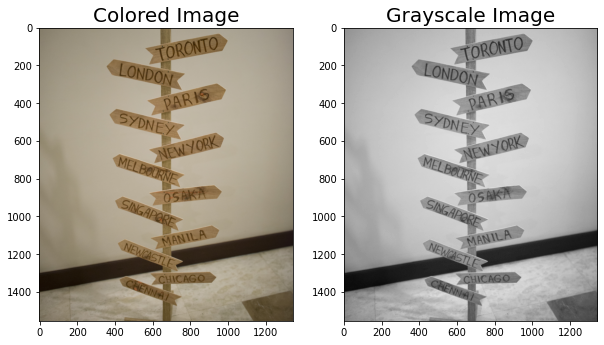Master LLMs with our FREE course in collaboration with Activeloop & Intel Disruptor Initiative. Join now!

## PublicationComputer Vision

# Image Processing using Spatial Filters

Last Updated on February 5, 2021 by Editorial Team

#### Giving Space…

One of the wonders of image processing is being able to see the pixel values of each image and perform different mathematical operations on it.

Since the image is already an array or a matrix, then we can already perform different operations like multiplying, adding, subtracting, and dividing.

One of the most used operation in image processing is convolution, which is primarily used for filtering the image.

The process usually stems from convolving an image array into a user-defined array or matrix.

This user-defined matrix are known as kernels and are usually have a different effect depending on the value they have especially the sign orientation,

For this article, we will try different spatial kernels that can convolve into a specific image.

Let us load our sample image!

`import numpy as npfrom skimage.io import imshow, imreadimport matplotlib.pyplot as plt`
`mple = imread('stand.png')imshow(sample);`

Using Different Filters:

Horizontal Sobel

`import matplotlib.pyplot as pltfrom scipy.signal import convolve2dimport numpy as np`
`sample_g = rgb2gray(sample)#defining the kernels/filter# Horizontal Sobel Filterkernel1 = np.array([[1, 2, 1],                    [0, 0, 0],                    [-1, -2, -1]])`
`conv1 = convolve2d(sample_g, kernel1, 'valid')fig, ax = plt.subplots(1,3,figsize=(15,10))ax.imshow(sample_g,cmap='gray')ax.imshow(kernel1,cmap='gray')ax.imshow(abs(conv1),cmap='gray')`
`ax.set_title('Grayscale Image',fontsize=20)ax.set_title('Filter Specs',fontsize=20)ax.set_title('Using Horizontal Sobel',fontsize=20)plt.show()`

The general step to convolve a separate array to another is to make the image is on the grayscale dimension. We can use a usable function from the scipy library to convolve a specific image. The codes for the following other filters follow some principle, the only thing that changes are the value of the array integers, this can be described by the filter specs graph.

The results show that the horizontal Sobel filter was able to attenuate the horizontal features and horizontal lines in the image.

Using Vertical Sobel

The experimental vertical Sobel was able to attenuate the vertical features of the image, for this example, we can see the vertical line of the stand more clearly if we compare it to the results of the horizontal Sobel enhancing.

Using Left Diagonal Filer

The diagonal filter in a sense is just the same as the vertical and horizontal Sobel but more attenuating the diagonal features of the image.

Using Right Diagonal Filter

We tried also doing the inverse of the left diagonal matrix which is the right diagonal matrix, and an interesting insight was seen, the results show that there is no difference between using the left or right diagonal filter.

Using Edge Detection Filter

As the name suggests, an edge detection filter is an array that can attenuate the edges of the image as from the results, we were able to clearly show the edges of the wooden stand.

Using a Sharpening Filter

Sharpening is always used in a lot of photo editing, and the math behind it is convolving an image to a predefined array wherein we can attenuate the center by putting negative values to the cross-segment.

Using a Box Blur Filter

Blurring is the act of subdividing the pixel values of the defined array. From the results, we might think that the image was not blurred, but in reality, is blurred by a fraction.

Using a Gaussian Blur Filter

Gaussian Blur Filter works the same way as the box blur but with a much more blurring effect

### Summary

In summary, we were able to show the different spatial filters that we can use for an image. Normally these filters are already built-in in a lot of photo editing software but the theory behind it is that all of the filters are stemmed from basic linear algebra functions.

Stay tuned for the next article!Image Processing using Spatial Filters was originally published in Towards AI on Medium, where people are continuing the conversation by highlighting and responding to this story.

Published via Towards AI